# Test: Three Dimensional 3D Geometry (Competition Level) - 2

## 30 Questions MCQ Test Mathematics (Maths) Class 12 | Test: Three Dimensional 3D Geometry (Competition Level) - 2

Description
This mock test of Test: Three Dimensional 3D Geometry (Competition Level) - 2 for JEE helps you for every JEE entrance exam. This contains 30 Multiple Choice Questions for JEE Test: Three Dimensional 3D Geometry (Competition Level) - 2 (mcq) to study with solutions a complete question bank. The solved questions answers in this Test: Three Dimensional 3D Geometry (Competition Level) - 2 quiz give you a good mix of easy questions and tough questions. JEE students definitely take this Test: Three Dimensional 3D Geometry (Competition Level) - 2 exercise for a better result in the exam. You can find other Test: Three Dimensional 3D Geometry (Competition Level) - 2 extra questions, long questions & short questions for JEE on EduRev as well by searching above.
QUESTION: 1

### If the distance of the point P(1, -2, 1) from the plane x + 2y - 2z = a, where a > 0 is 5, then foot of perpendicular from P to the plane is

Solution:

Distance of pt P from plane = 5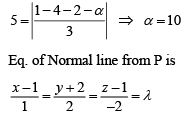Gen. pt. on line is (λ + 1, 2λ - 2, -2λ + 1)
This pt line on plane
(λ + 1 + 2 2λ - 2) - 2 (-2λ + 1) = 10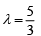QUESTION: 2

### The image of point (3, -2, 1) in plane 3x – y + 4z = 2 is

Solution:

Let Q be the image of point P(3, -2, 1) PQ is normal to the plane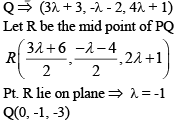QUESTION: 3

### The equation of plane passing through the point (0, 7, -7) and containing the line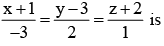Solution:

The equation of plane passing through (0, 7, -7) is a(x – 0) + b(y – 7) + c(z + 7) = 0
Plane contains line and passes through (-1, 3, -2)
∴ a(-1) + b(3 – 7) + c(-2 + 7) = 0
-3a + 2b + c = 0
∴ a : b : c = 1 : 1 : 1
x + y + z = 0

QUESTION: 4

Let the line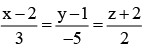lie in the plane x + 3y - az + b = 0, then (a, b) equals

Solution:

Line is perpendicular to the normal of plane
3 × 1 + (-5) × 3 + 2 (-α) = 0
– 12 – 2α = 0
α = – 6
Point (2, 1, -2) lie on plane
∴ b = 7

QUESTION: 5

The angle between line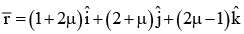and plane 3x – 2y + 6z = 0 is (m is scalar)

Solution:

D. R’s of line are 2 : 1 : 2
D.R’s of Normal to plane is :3 : -2 : 6 Angle between line and plane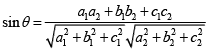QUESTION: 6

Let P(3, 2, 6) be a point in space and Q be a point on line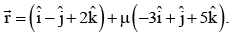Then value of m for which the vector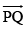is parallel to the plane x-4y + 3z = 1 is

Solution: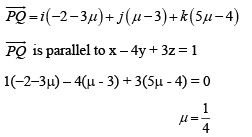QUESTION: 7

A variable plane at a distance of 1 unit from the origin cut the co-ordinate axis at A, B and C. If the centroid D (x, y, z) of ΔABC satisfy the relation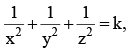then value of k is

Solution:

Equation of plane in intercept form is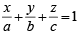Centroid of ΔABC(a/3, b/3, c/3)
It satisfies the relation
∴ 9/a2 + 9/b2 + 9/c2 = k
Perpendicular distance is 1 unit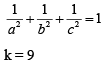QUESTION: 8

The Cartesian equation of plane passing through (1, 1, 1) and containing the x-axis is

Solution:

Eq. of x-axis is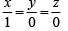Equation of plane passing through (1, 1, 1) and containing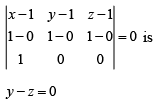QUESTION: 9

If the plane 2x – y + z = 0 is parallel to the line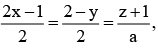then value of a
is

Solution:

Plane is parallel to line i.e., normal of plane is perpendicular to the line
2 × 1 + (-1) × (-2) + 1 × a = 0
2 + 2 + a = 0
a = - 4

QUESTION: 10

Let L be the line of intersection of planes 2x + 3y + z = 1 and x + 3y + 2z = 2. If L makes an angle ‘α’ with the positive x-axis, then cosa equals

Solution:

Let the direction cosines of line be
l, m, n then
2l + 3m + n = 0
And l + 3m + 2n = 0
On solving, l : m : n = 1 : - 1 : 1
l2 + m2 + n2 = 1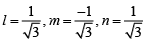Line L make an angle ‘α’ with +ve x-axis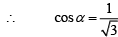QUESTION: 11

The ratio in which the line joining (2, 4, 5), (3, 5, -4) is divided by the yz-plane is

Solution:

Let A(2, 4, 5) and B(3, 5, -4) and C be the point in which line AB meets the yz-plane. Let C divide AB in the ratio k : 1, then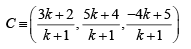But C lies on yz-plane, therefore,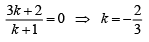QUESTION: 12

The equation of the plane through the point (-1, 2, 0) and parallel to the line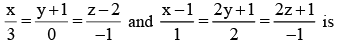Solution:

P(-1,2,0) = -i + 2j
L1 = x/3 = (y+1)/0 = (z-2)/(-1)
a = 3i + 0j - k
L2 = (x-1)/1 = 2(y+½)/2 = 2(z+½)/(-1)
b = i + j - k/2
Plane is parallel to a  ⇒ n is perpendicular to a
Plane is parallel to b  ⇒ n is perpendicular to b
n = a * b = {(3, 0, -1) (1,1, -½)}
= i(0+1) -j(-3/2+1) +k(3-0)
= i + 1/2j + 3k
PR = R - P = (x+1)i + (y-z)j + zk
PR. n = 0
(x+1)*1 + (y-z)*½ + z*3 = 0
x+1 + y/2 - 1 + 3z = 0
x + y/2 + 3z = 0
2x + y + 6z = 0

QUESTION: 13

Equation of the line through (1, 1, 1) and perpendicular to the plane 2x + 3y – z – 5 = 0 is

Solution:

DR’s of the normal to the given plane are < 2, 3, -1 >.
Hence, the required line is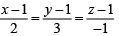QUESTION: 14

Let A (1,1,1) , B (2, 3, 5) and C (-1, 0, 2) be three points, then equation of a plane parallel to the plane ABC which is a distance 2 is

Solution:

A (1,1,1), B (2,3,5), C(-1,0,2) direction ratios of AB are < 1,2,4>
Therefore, direction ratios of normal to plane ABC are <2, -3,1>
As a result, equation of the required plane is 2x – 3y +z = k then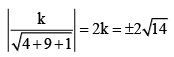Hence, equation of the required plane is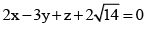QUESTION: 15

The distance of the point (2, 1, -1) from the plane x - 2y + 4z = 9 is

Solution:

Required distance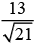QUESTION: 16

​A variable plane is at a constant distance 3p form the origin and meets the axes in A, B and C. The locus of the centroid of the triangle ABC is

Solution: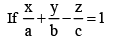Distance from origin = 3pQUESTION: 17

The angle between the straight line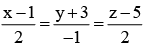and the plane 4x - 2y - 5z = 9
is

Solution:

Explanation:-  (x-1)/2=(y+3)/(-1)=(z-5)/2

4x - 2y - 5z = 9

cosθ=(2*4 - 1*2 + 2*(-5))/(3)√45)=0

= (8 - 2 -10)/3√45

= -4/(9√5)

Hence, angle between normal to plane and given line is 90°

QUESTION: 18

The distance between the line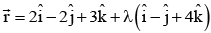and the plane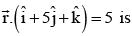Solution: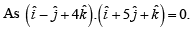Therefore, the line and the plane are parallel. A point on the line is (2, -2, 3). Required distance = distance of (2, -2, 3) from the given plane x + 5y + z - 5 = 0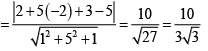QUESTION: 19

Equation of the plane containing the straight line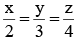and perpendicular to the
plane containing the straight line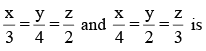Solution:

The Dr’s to normal of the plane containing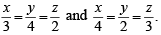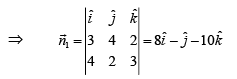Let required plane is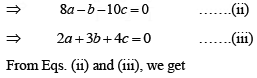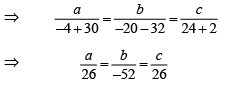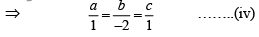From Eqs. (i) and (iv), required equation of plane, is x - 2 y + z =0

QUESTION: 20

If the distance between the plane x - 2y + z = d and the plane containing the line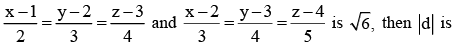Solution:

Equation of plane containing the given lines is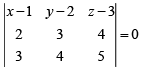⇒ (x -1)(-1) - (y - 2)(-2) + (z - 3)(-1) = 0
⇒ -x +1+ 2y - 4 - z + 3 = 0
⇒ -x + 2 y - z = 0......(i)
Given plane is
x - 2 y + z = d .......(ii)
Eqs. (i) and (ii) are parallel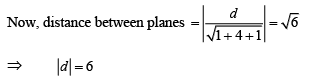QUESTION: 21

If the lines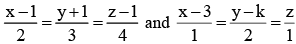intersect, then k is equal to

Solution:

Point of the first line (2t + 1, 3t - 1, 4t + 1)
Point of the second line (s + 3, 2s+ k, s) by comparing the points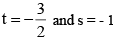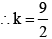QUESTION: 22

A line with positive direction cosines passes through the point P(2, -1, 2) and makes equal angle with the coordinate axes. The line meets the plane 2x + y + z = 9 at point Q. The length of the line segment PQ equals

Solution: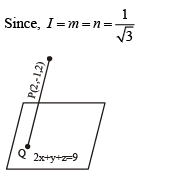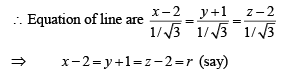∴ Any point on the line is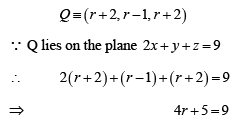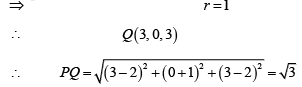QUESTION: 23

The d.r’s of the line AB are 6, - 2,9. If the line AB makes angles α,β with oy, oz respectively where O = ( 0, 0, 0) then sin2α - sin2β =

Solution:

Explanation:- Given, DR's of

AB =(6,−2,9)

cosα=(AB.i^)/|AB||i^|

= [6^2+2^2+9^2]^1/2

= 6/11

cosβ=−2/11

cosγ= 9/11

sin^2α−sin^2β=(1−cos^2α)−(1−cos^2β)

=cos^2β−cos^2α

=(4)/121 − (36)/121

​= (−32)/121

QUESTION: 24

A line makes the same angle θ with each of the x - axis and z - axis. It makes β angle with y - axis such that sin2 β = 3sin2 θ then cos2 θ

Solution: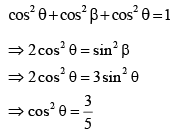QUESTION: 25

If the angle θ between the line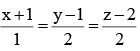and plane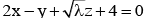is such that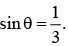Then, value of λ is

Solution: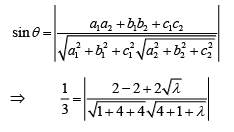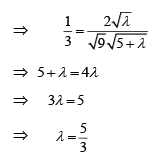QUESTION: 26

The line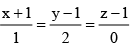Solution:

Direction cosines of the line are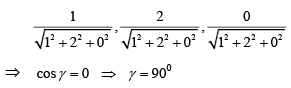⇒ (A) is correct

QUESTION: 27

A plane π passes through the point (1, 1, 1). If b, c, a are the direction ratios of a normal to the plane, where a, b, c ( a < b< c ) are the prime factors of 2001, then the equation of the plane π is

Solution: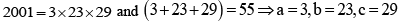QUESTION: 28

The point of intersection of the lines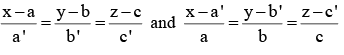must be

Solution: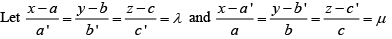As the two lines intersect, we must have
a + a 'λ = a '+ μa,b + b 'λ = b '+μb and c + c 'λ = c'+ cμ
Solving a 'λ -μa = a'- a and b 'λ -μb = b'- b
We get, μ = 1 and λ = 1
⇒ x = a + a', y = b +b' and z = c+ c'
∴ Point of intersection is ( a + a ', b + b ', c + c ')
⇒ (B) is correct

QUESTION: 29

The direction ratios of a normal to the plane through (1, 0, 0), (0, 1, 0) which makes an angle of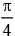with the plane x + y = 3 are

Solution:

Any plane through (1,0,0) is
A (x -1) + B (y - 0) + C (z - 0) = 0 ..(1)
It contains (0,1,0) if -A + B = 0.... (2)
Also (1) makes an angle ofwith the plane x + y= 3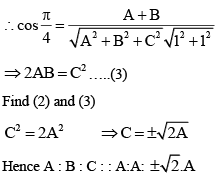QUESTION: 30

The equation of the plane passing through the mid-point of the line joining the points (1, 2, 3) and (3, 4, 5) and perpendicular to it is:

Solution:

Direction ratio of line joining (1, 2, 3) and (3, 4, 5) are (2, 2, 2).
⇒ Normal to the plane has DR’s (2, 2, 2)
Now plane passes through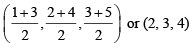⇒ Equation of plane is
2(x – 2) + 2(y – 3) + 2(z – 4) = 0
x + y + z = 9
⇒ (A) is correct